# TriangulateMesh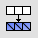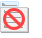The TriangulateMesh command splits all planar quadrangular polygon mesh faces into two triangular mesh faces.

# TriangulateRenderMeshes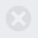The TriangulateRenderMeshes command splits all planar quadrangular polygon render mesh faces into two triangular mesh faces.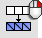Mesh

Edit Tools >

The TriangulateNonPlanarQuads command splits all non-planar quadrangular polygon mesh faces into two triangular mesh faces.

Options

##### Distance

Any quadrangle where the distance of the quad's fourth point from the plane defined by the quad's first three points is greater than or equal to the Distance value will be triangulated.

##### Angle

Any quadrangle where the angle between the plane normals of the face triangles if it were triangulated is greater than or equal to the Angle value will be triangulated.

##### Both

Use both distance and angle criteria.

##### Increment

The value by which the Distance and Angle edit boxes are increased or decreased with the spinner controls.

Select a quadrangular mesh face to set the Distance and Angle values.

##### Shortest diagonal

Divide along the short diagonal.

##### Longest diagonal

Divide along the long diagonal.

##### Minimize area

Minimize resulting area.

##### Maximize area

Maximize resulting area.

##### Minimum angle

Minimize angle between triangle normals.

##### Maximum angle

Maximize angle between triangle normals.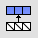Diagonals that are unwelded (have unique vertexes at the corners) will not be considered as candidates for merging triangles into quadrangles.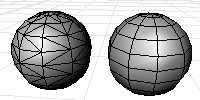#### Steps

Command-line options

Planarity

The angle between the face normals of the triangles.

Rectangularity

Specify a number greater than or equal to 1. If the adjacent triangles pass the planarity test then they will be merged into a quadrangle if the largest ratio of the lengths of the diagonal is less than or equal to the number you typed, then the triangles are converted to quadrangle.

Increment

Increment value used by the spinner controls.